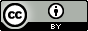Minimality exercise from Halmos

Status: notes

The task given in Halmos’s Naive Set Theory is to show that given some subset of a natural number $$E$$, there is some $$k\in E$$ such that for any other $$m\in E$$, we have $$k\in m$$. Intuitively, we know that “$$k\in m$$” means “$$k < m$$”, but since we haven’t defined order yet in the book, we have to deal just with the set theoretic properties of the naturalvnumbers. Now how should we go about finding this $$k$$? One way is to look at an example to see what happens. Suppose we take $$E = \{1,3,7\}$$. Then the “$$k$$” that we want is $$1$$, since $$1\in 3, 1\in 7$$.